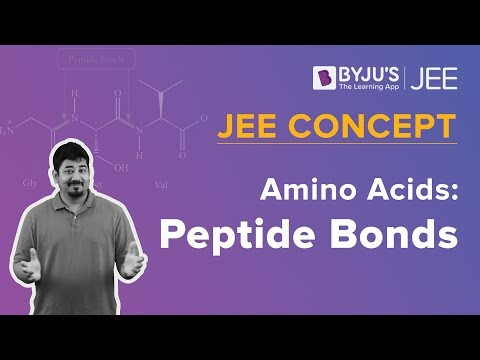Win up to 100% scholarship on Aakash BYJU'S JEE/NEET courses with ABNAT Win up to 100% scholarship on Aakash BYJU'S JEE/NEET courses with ABNAT

# Biomolecules – JEE Advanced Previous Year Questions with Solutions

Biomolecules JEE Advanced Questions with Solutions are provided here to help JEE aspirants take a look at some of the previous year questions and understand the question types, difficulty level and important topics. Additionally, the solutions that we have provided here will enable students to prepare in the right way. We have tried to use very simple and straightforward language to help each learner understand the concepts covered in the questions in a clear manner.

JEE aspirants can also download Biomolecules JEE Advanced Questions with Solutions PDF for free and make a self-assessment by solving the questions given in this chapter. Alternatively, solving the questions and going through the solutions will not only enhance the student’s grip on the topic but they will also develop better problem-solving skills and be ready to face any type of questions that may be asked in the entrance exam.

## JEE Advanced Previous Year Questions on Biomolecules

Question 1. Which of the following statement(s) is(are) true?

A. Hydrolysis of sucrose gives dextrorotatory glucose and laevorotatory fructose

B. Oxidation of glucose with bromine water gives glutamic acid

C. The two six-membered cyclic hemiacetal forms of D-(+)-glucose are called anomers

D. Monosaccharides cannot be hydrolysed to give polyhydroxy aldehydes and ketones

Solution: (A, C and D)

α − D − glucopyranose and β − D − glucopyranose are anomers of each other.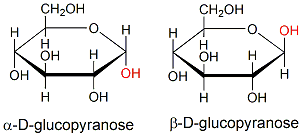A carbohydrate that cannot be hydrolyzed further to give simpler units of polyhydroxy aldehyde or ketone is called a monosaccharide.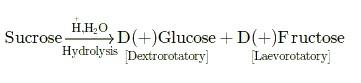Sucrose is dextrorotatory in nature and on hydrolysis it gives dextrorotatory glucose and laevorotatory fructose.

Question 2. The Fischer presentation of D-glucose is given below.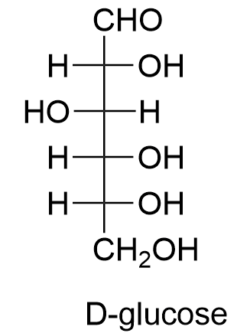The correct structure(s) of β-L-glucopyranose is (are):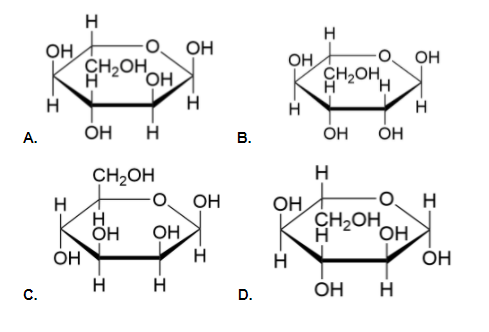Solution: (D)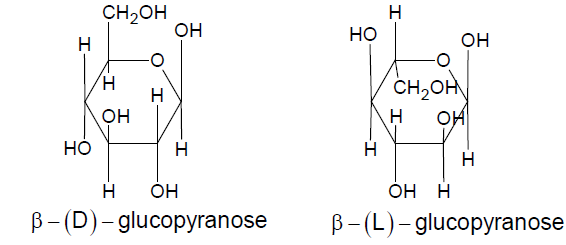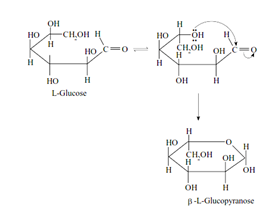Question 3. For ‘invert sugar’, the correct statement(s) is (are).

(Given: specific rotations of (+)-sucrose, (+)-maltose, L-(−)-glucose and L-(+)-fructose in aqueous solution are +66o,+140o,−52o and +92o, respectively)

(A) ‘invert sugar’ is prepared by acid-catalyzed hydrolysis of maltose

(B) ‘invert sugar’ is an equimolar mixture of D-(+) glucose and D-(–)-fructose

(C) The specific rotation of ‘invert sugar is -20o

(D) On reaction with Br2 water, ‘invert sugar’ forms saccharic acid as one of the products

Solution: (B, C)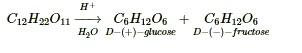Sucrose Hydrolysis →D(+)− glucose [α] =(−52o) + D(−) fructose [α] = (+92o)

∴ α invert sugar = + 52o + (-92o) / 2 = -20o

Question 4. The structure of D−(+)-glucose is given as.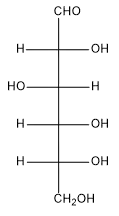The structure of L−(−)-glucose is: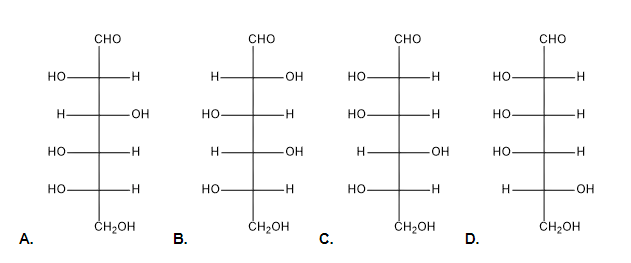Solution: (A)

The structures of D(+)-glucose and L(-)-glucose are shown below. They mostly differ in configuration at second, third, fourth and fifth C atoms.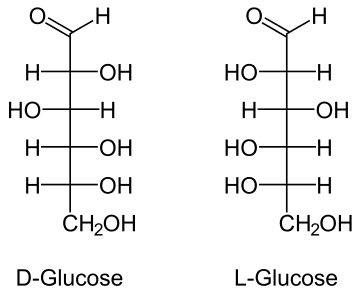Question 5. The structure of a peptide is given below.

If the absolute values of the net charge of the peptide at pH = 2, pH = 6, and pH = 11 are | 1|, | 2|, and | 3|, respectively, then what is | 1| + | 2| + | 3|?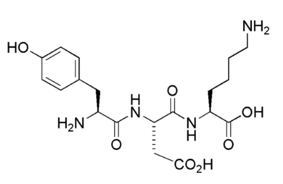Solution: (5)

Therefore ∣z1∣ + ∣z2∣ + ∣z3​∣ = 2 + 0 + 3 = 5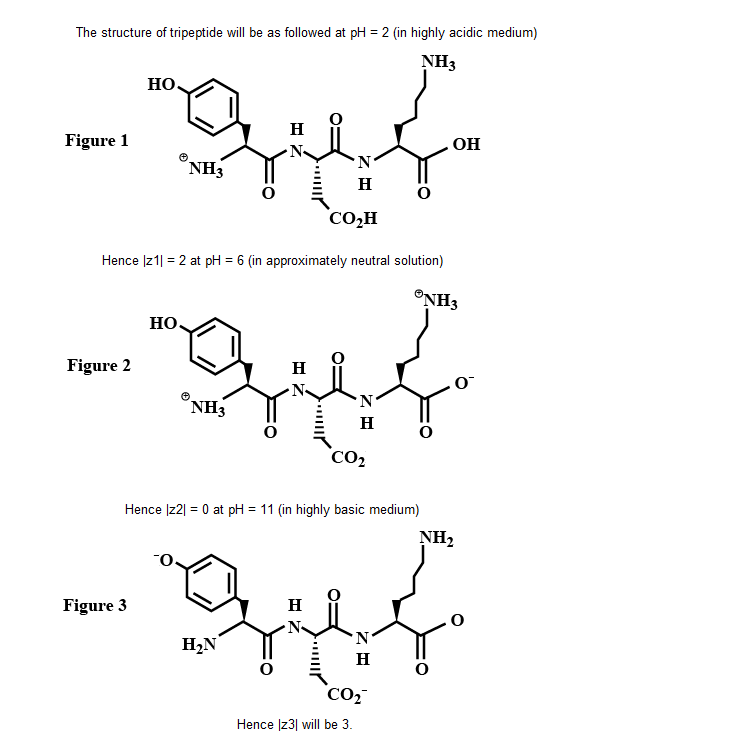Question: 6. The total number of distinct naturally occurring amino acids obtained by complete acidic hydrolysis of the peptide shown below is;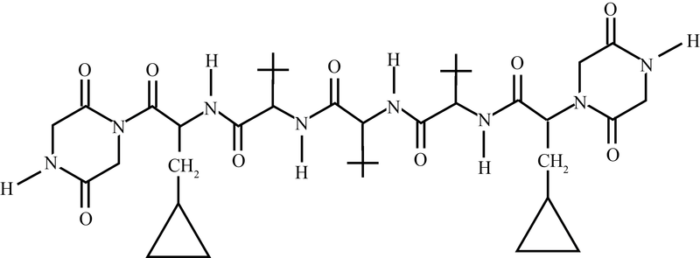A. 1

B. 2

C. 3

D. 4

Solution: (A)

Here, we basically have to calculate the total number of naturally occurring amino acids when the peptide undergoes acidic hydrolysis.

This problem can be solved by performing hydrolysis of peptides and deciding the nature of the product. Chemical reaction and product formed after hydrolysis of the given peptide can be represented as is glycine which is only naturally occurring amino acid. While (B) (C) and (D) are not naturally occurring amino acids. Hence, the correct integer is (1).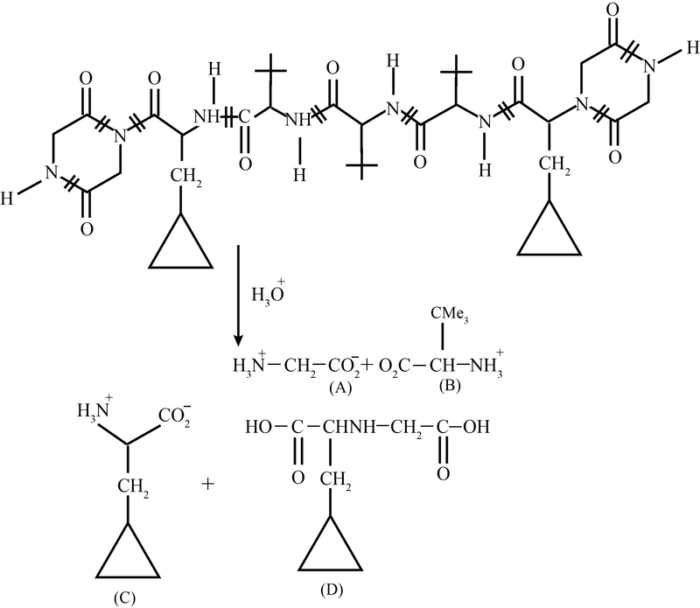Question 7. A tetrapeptide has a -COOH group on alanine. This produces glycine (Gly), valine (Val), phenylalanine (Phe) and alanine (Ala), on complete hydrolysis. For this tetrapeptide, the number of possible sequences (Primary structures) with – NH2 group attached to a chiral centre is___

A. 12

B. 6

C. 4

D. None of these

Solution: (C)

As mentioned in the question the −COOH group is attached to alanine wherein one terminal of the tetrapeptide contains alanine. By this, we understand that its position is fixed.

Meanwhile, Glycine is achiral and valine and phenylalanine are chiral. Therefore, the −NH2 group is attached to either valine or phenylalanine. The following are the four possible combinations of the tetrapeptide.

H2N−Val−Gly−Phe−Ala−COOH

H2N−Val−Phe−Gly−Ala−COOH

H2N−Phe−Gly−Val−Ala−COOH

H2N−Phe−Val−Gly−Ala−COOH

Therefore, we can consider A as the correct option.

Question 8. The substituents R1 and R2 for nine peptides are listed in the table given below. How many of these peptides are positively charged at pH = 7.0?

Peptide R1 R2
I H H
II H CH3
III CH2COOH H
IV CH3CONH2 (CH2)4NH2
V CH2CONH2 CH2CONH2
VI (CH2)4NH2 (CH2)4NH2
VII CH2COOH CH2CONH2
VIII CH2OH (CH2)4NH2
IX (CH2)4NH2 CH3

A. 4

B. 6

C. 8

D. 9

Solution: (A)

The amino acid remains completely in Zwitterionic at its isoelectric point. Amino acids with the additional acidic groups have then isoelectric pH of less than 7.0 and increasing pH above the isoelectric point makes them anionic.

On the other hand, amino acids with the additional basic group have their isoelectric pH greater than 7.0 and decreasing pH below the isoelectric point (by adding acid solution) makes them cationic. The given peptide with the following R1 and R2 are basic, will remain protonated (cationic) at pH = 7.0.

Thus, 4 is the correct integer.

Question 9. When the following aldohexose exists in its D-configuration, the total number of stereoisomers in its pyranose form is (numeral) ___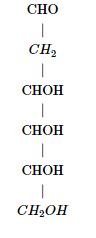Solution: (8)

The D-form of given sugar is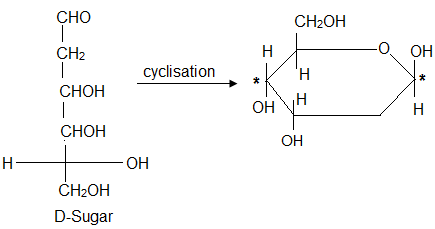Hence, there are three chiral carbon atoms.

∴ The total number of stereoisomers of D-pyranose is 23 = 8

Question 10. The total number of basic groups in the following form of lysine is.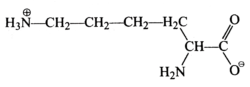Solution: (2)

0 and NH2 are two basic groups in lysine.

## Biomolecules and Polymers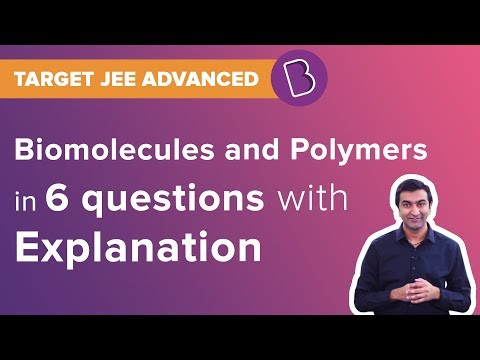## Peptide Bonds